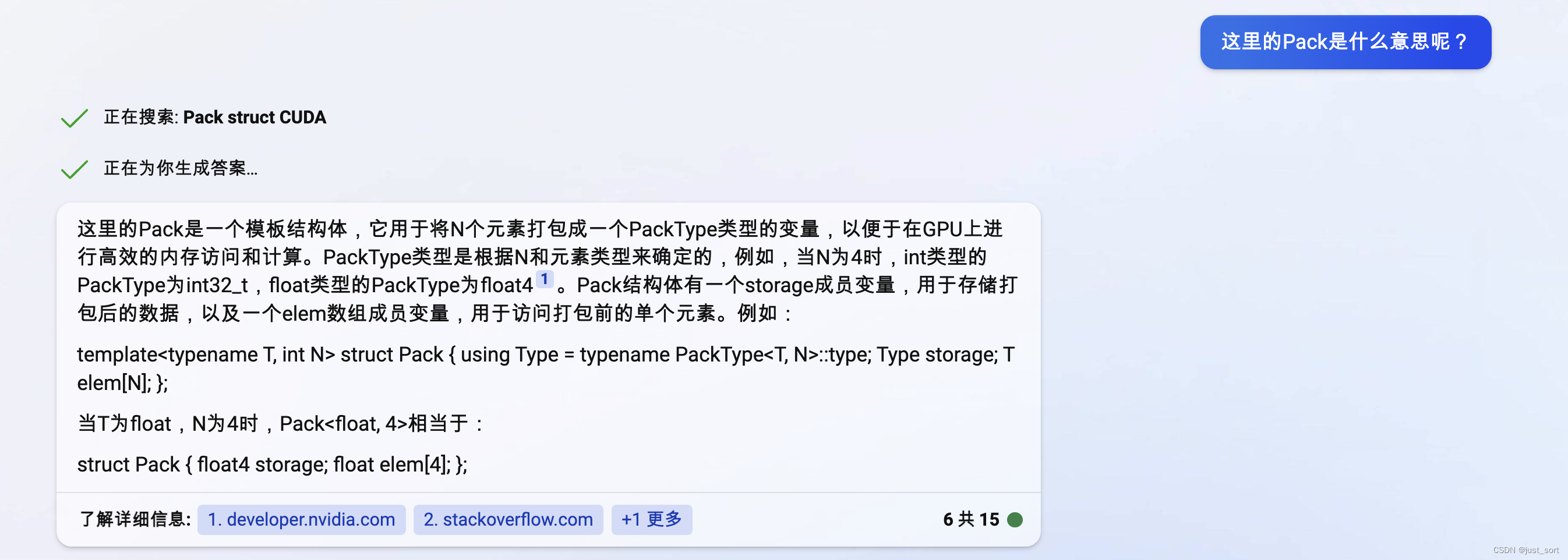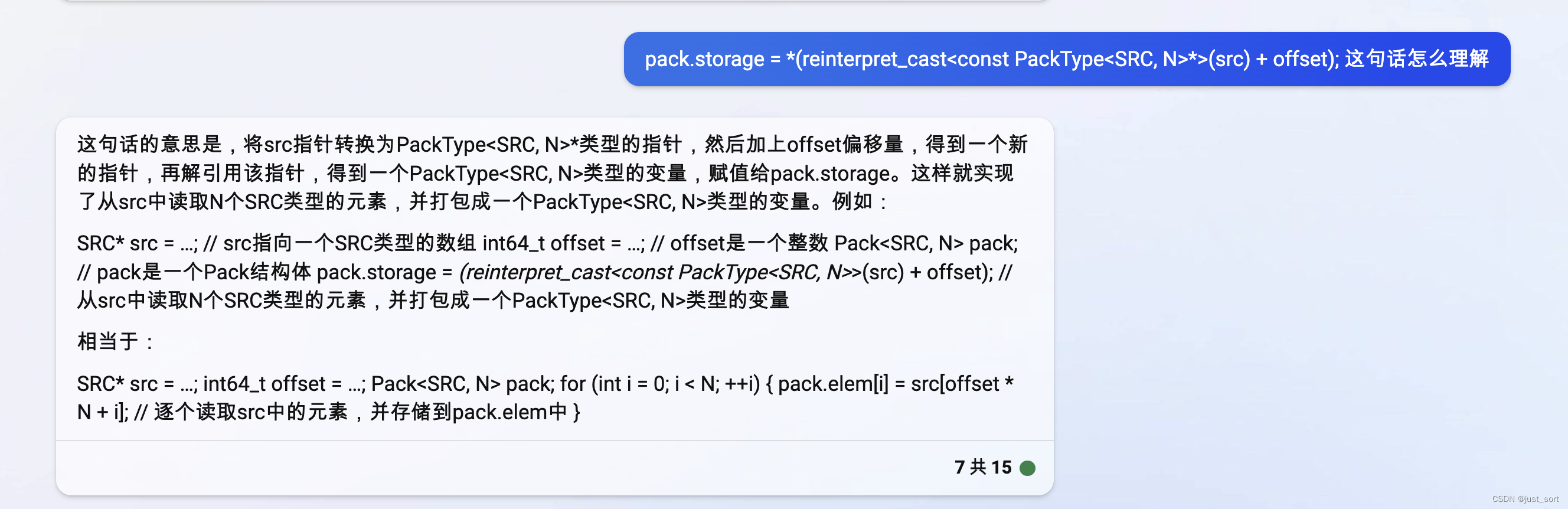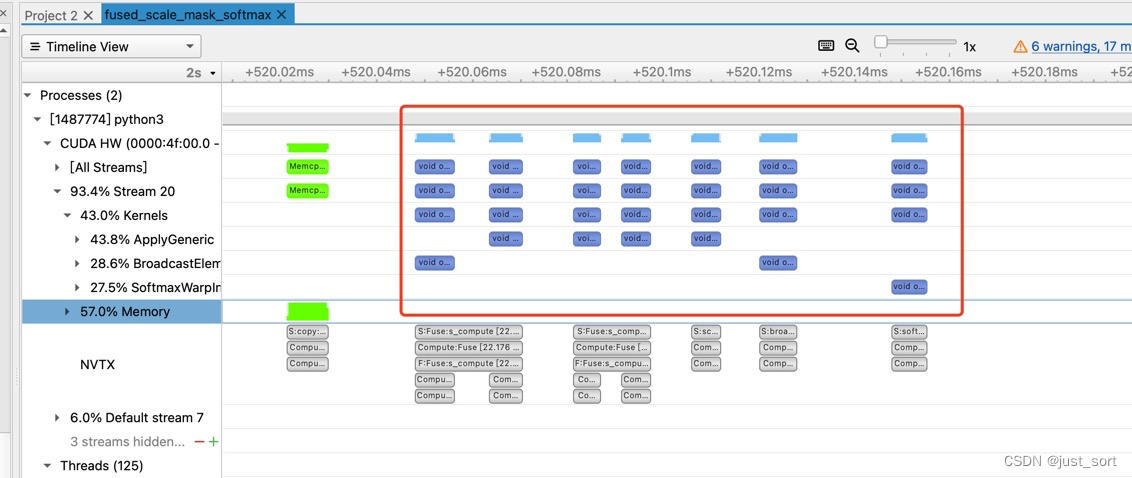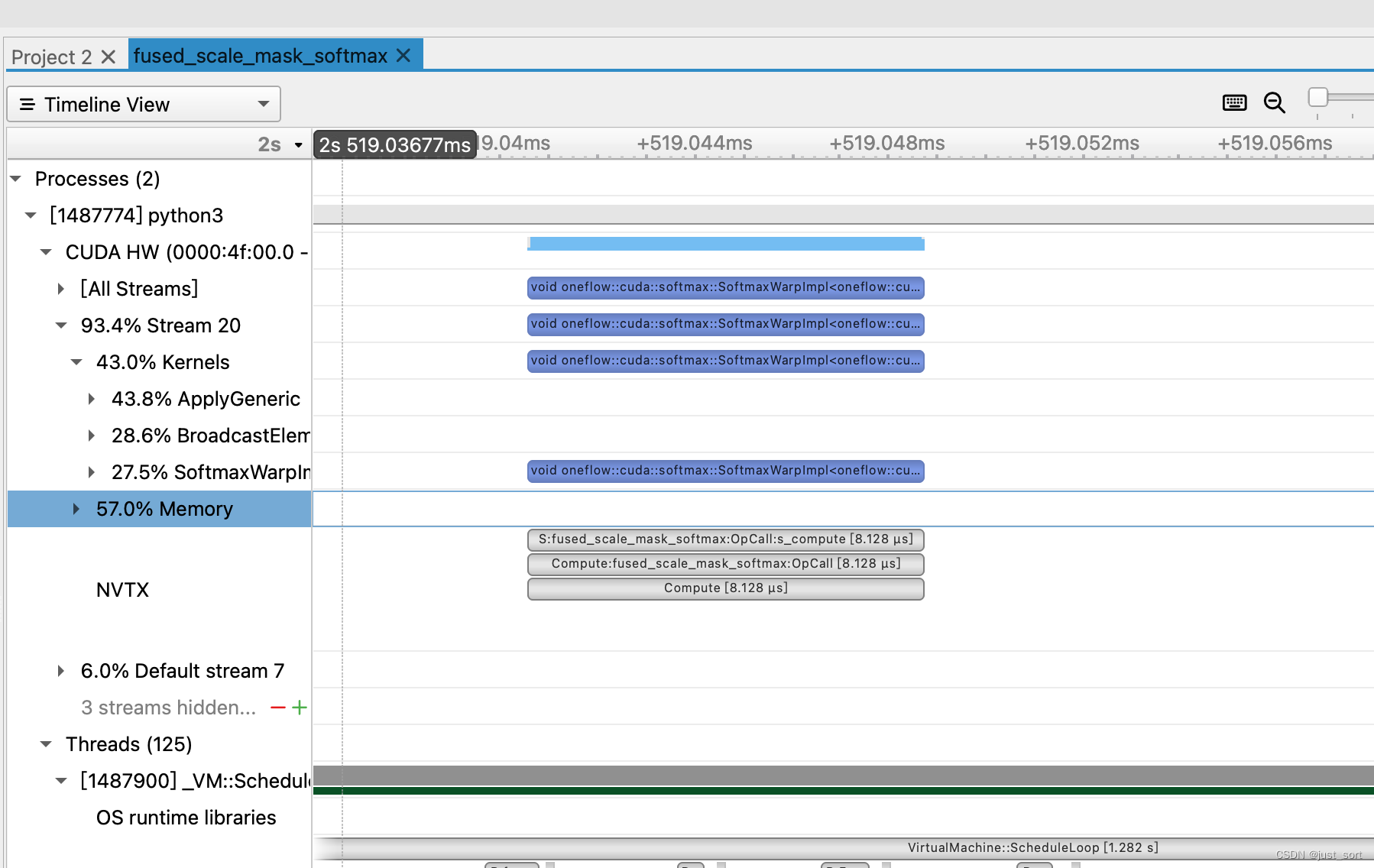# 0x1. Pattern介绍¶

import numpy as np
import oneflow as flow

batch_size = 4
seq_length = 64
fill_value = -10000.0
scale_value = 2.0

x = np.random.randn(batch_size, num_heads, seq_length, seq_length).astype(
np.float32
)

fused_x_tensor = flow.tensor(x, dtype=flow.float32).to("cuda")

)

origin_x_tensor = flow.tensor(x).to("cuda")
origin_out = flow.mul(
) * scale_value + fill_value * (1.0 - origin_mask_tensor)
origin_out = flow.softmax(origin_out, dim=-1)
assert(np.allclose(fused_out.numpy(), origin_out.numpy(), atol=1e-4, rtol=1e-4))


# 0x2. 使用chatgpt来读代码实现¶

template<typename SRC, typename DST, typename MASK>
template<int N>
__device__ void load(DST* dst, int64_t row, int64_t col) {
Pack<SRC, N> pack;
const int64_t offset = (row * param.row_size + col) / N;
pack.storage = *(reinterpret_cast<const PackType<SRC, N>*>(src) + offset);
#pragma unroll
for (int i = 0; i < N; ++i) {
dst[i] = static_cast<DST>(param.fill);
} else {
dst[i] = static_cast<DST>(pack.elem[i]) * static_cast<DST>(param.scale);
}
}
}
const SRC* src;
};


// 它的功能是定义一个模板类，用于在kernel函数中加载逐元素掩码softmax的输入。它的模板参数有：
// SRC: 源张量的数据类型
// DST: 目标张量的数据类型
// 它的构造函数接受以下参数：
// src: 源张量的指针
// dst: 目标张量的指针
// row: 当前的行号
// col: 当前的列号
// 它的内部逻辑是：
// 根据行号和列号计算源张量和掩码张量的偏移量
// 遍历N个元素，如果掩码张量的元素为0，就将目标张量的元素设为填充值，否则就将源张量的元素乘以缩放因子，并转换为目标张量的数据类型
// 这个模板类的成员变量有：
// src: 源张量的指针
// 这个模板类的目的是为了方便在kernel函数中使用逐元素掩码softmax的输入，提高深度学习的性能。我们再问一下这里的Pack是什么意思？可以看到chatgpt还是较为准备的为我们解释了这里Pack的含义以及指出是便于在GPU上进行高效的内存访问。// 它的功能是定义一个模板类，用于在内核函数中加载广播掩码softmax的输入。它的模板参数有：
// SRC: 源张量的数据类型
// DST: 目标张量的数据类型
// num_dims: 张量的维度数
// IndexType: 索引的数据类型
// 它的构造函数接受以下参数：
// src: 源张量的指针
// 它的成员函数有：
// dst: 目标张量的指针
// row: 当前的行号
// col: 当前的列号
// 它的内部逻辑是：
// 根据行号和列号计算源张量的偏移量
// 根据偏移量计算源张量的多维索引
// 根据多维索引计算掩码张量的偏移量
// 遍历N个元素，如果掩码张量的元素为0，就将目标张量的元素设为填充值，否则就将源张量的元素乘以缩放因子，并转换为目标张量的数据类型
// 这个模板类的成员变量有：
// src: 源张量的指针
// 这个模板类的目的是为了方便在内核函数中使用广播掩码softmax的输入，提高深度学习的性能。
template<typename SRC, typename DST, typename MASK, size_t num_dims, typename IndexType>
for (int i = 0; i < num_dims; i++) { mask_dims[i] = params.mask_dims[i]; }
}
template<int N>
__device__ void load(DST* dst, int64_t row, int64_t col) {
Pack<SRC, N> pack;
const IndexType offset = row * params.row_size + col;
IndexType input_index[num_dims];
params.src_index_helper.OffsetToNdIndex(offset, input_index);
for (int dim = 0; dim < num_dims; ++dim) {
} else {
}
}
pack.storage = *(reinterpret_cast<const PackType<SRC, N>*>(src) + offset / N);
#pragma unroll
for (int i = 0; i < N; ++i) {
dst[i] = static_cast<DST>(params.fill);
} else {
dst[i] = static_cast<DST>(pack.elem[i]) * static_cast<DST>(params.scale);
}
}
}
const SRC* src;
};


// 它的功能是定义一个结构体，用于存储广播掩码softmax的参数。
// 广播掩码softmax是一种在不同形状的张量之间进行softmax运算的方法，
// 它会将较小的张量扩展到较大的张量的形状上，并用一个掩码张量来指定哪些位置需要计算softmax，
// 哪些位置需要填充一个固定的值。这个结构体的成员变量有：
// src_index_helper: 一个用于计算源张量索引的辅助类
// row_size: 每一行的元素个数
// fill: 填充的值
// scale: softmax的缩放因子
// 这个结构体的目的是为了方便在内核函数中使用广播掩码softmax的参数，提高深度学习的性能。
template<size_t num_dims, typename IndexType>
NdIndexOffsetHelper<IndexType, num_dims> src_index_helper;
int64_t row_size;
float fill;
float scale;
};


// 定义 NdIndexOffsetHelper 类来做多维张量的坐标映射
template<typename T, int N>
class NdIndexOffsetHelper {
public:
NdIndexOffsetHelper() = default;

// 这段代码是一个构造函数模板，它的功能是根据给定的多维数组的维度初始化一个用于计算偏移量的辅助类。
// 它的参数是一个可变参数模板，表示可以接受任意个数和类型的参数，但是第一个参数必须是一个T类型的值，
// 后面的参数必须是一个参数包。它的函数体是一个调用另一个函数的语句，这个函数是用于初始化步长数组的。
// 它使用了一个宏OF_DEVICE_FUNC，这个宏可能是用于指定函数在哪个设备上运行的，比如GPU或者CPU。
// 这个构造函数模板可能是用于实现一些多维数组的操作，比如插值或者转置。
template<class... Ts>
OF_DEVICE_FUNC explicit NdIndexOffsetHelper(T d0, Ts... dims) {
constexpr int n = 1 + sizeof...(dims);
static_assert(n <= N, "");
T dims_arr[n] = {d0, static_cast<T>(dims)...};
// 初始化strides信息
InitStrides(dims_arr, n);
}

// 从一个类型为T的数组进行构造，并初始化strides信息，注意这里的strides长度设置为N
OF_DEVICE_FUNC explicit NdIndexOffsetHelper(const T* dims) { InitStrides(dims, N); }

// 从一个类型为U的数组进行构造，并初始化strides信息，注意这里的strides长度设置为N
template<typename U>
OF_DEVICE_FUNC explicit NdIndexOffsetHelper(const U* dims) {
T dims_arr[N];
for (int i = 0; i < N; ++i) { dims_arr[i] = dims[i]; }
InitStrides(dims_arr, N);
}

// 从一个类型为T的数组进行构造，并初始化strides信息，注意这里的strides长度自定义为n
OF_DEVICE_FUNC explicit NdIndexOffsetHelper(const T* dims, int n) { InitStrides(dims, n); }

// 从一个类型为U的数组进行构造，并初始化strides信息，注意这里的strides长度自定义为n
template<typename U>
OF_DEVICE_FUNC explicit NdIndexOffsetHelper(const U* dims, int n) {
T dims_arr[N];
for (int i = 0; i < N; ++i) {
if (i < n) { dims_arr[i] = dims[i]; }
}
InitStrides(dims_arr, n);
}

// virtual 表示这是一个虚析构函数，用于在删除基类指针时调用派生类的析构函数，避免内存泄漏。
// ~NdIndexOffsetHelper() 表示这是 NdIndexOffsetHelper 类的析构函数，用于释放类对象占用的资源。
// = default; 表示这是一个默认的析构函数，没有自定义的操作，让编译器自动生成。
virtual ~NdIndexOffsetHelper() = default;

// 这段代码是一个模板函数，用于根据一个N维索引数组计算一个一维偏移量。函数的参数和返回值都是模板类型T，可以是任意数值类型。函数的主要步骤如下：
OF_DEVICE_FUNC T NdIndexToOffset(const T* index) const {
// 定义一个变量offset，初始值为0，用于存储最终的偏移量。
T offset = 0;
#ifdef __CUDA_ARCH__
#pragma unroll
#endif
// 使用一个循环，从0到N-1，遍历索引数组的每一个元素。在循环中，使用一个数组stride_，
// 用于存储每一个维度的步长，即每增加一个单位的索引，偏移量增加多少。
// 将索引数组的第i个元素乘以步长数组的第i个元素，然后累加到offset上。
for (int i = 0; i < N; ++i) { offset += index[i] * stride_[i]; }
return offset;
}

// 类似上面，不过这里是从0到n进行循环
OF_DEVICE_FUNC T NdIndexToOffset(const T* index, int n) const {
assert(n <= N);
T offset = 0;
#ifdef __CUDA_ARCH__
#pragma unroll
#endif
for (int i = 0; i < N; ++i) {
if (i < n) { offset += index[i] * stride_[i]; }
}
return offset;
}

// 这段代码是一个函数模板，它的功能是根据给定的多维索引计算一个一维偏移量。
// 它的参数是一个可变参数模板，表示可以接受任意个数和类型的参数，但是第一个
// 参数必须是一个T类型的值，后面的参数必须是一个参数包。它的返回值也是一个T类型的值。
// 它的函数体是一个循环，用于累加每个维度的索引乘以对应的步长，得到最终的偏移量。
// 它使用了一个宏OF_DEVICE_FUNC，这个宏可能是用于指定函数在哪个设备上运行的，
// 比如GPU或者CPU。这个函数模板可能是用于实现一些多维数组的操作，比如插值或者转置。
template<class... Ts>
OF_DEVICE_FUNC T NdIndexToOffset(T d0, Ts... others) const {
constexpr int n = 1 + sizeof...(others);
static_assert(n <= N, "");
T index[n] = {d0, others...};
T offset = 0;
#ifdef __CUDA_ARCH__
#pragma unroll
#endif
for (int i = 0; i < n - 1; ++i) { offset += index[i] * stride_[i]; }
if (n == N) {
offset += index[n - 1];
} else {
offset += index[n - 1] * stride_[n - 1];
}
return offset;
}

// 这段代码是一个成员函数模板，它的功能是根据给定的一维偏移量计算一个多维索引。
// 它的参数是一个T类型的值，表示偏移量，和一个T类型的指针，表示索引数组。
// 它的函数体是一个循环，用于逐个维度地计算索引值，然后更新剩余的偏移量。
// 它使用了一个宏OF_DEVICE_FUNC，这个宏可能是用于指定函数在哪个设备上运行的，比如GPU或者CPU 。
// 这个成员函数模板可能是用于实现一些多维数组的操作，比如插值或者转置。
OF_DEVICE_FUNC void OffsetToNdIndex(T offset, T* index) const {
T remaining = offset;
#ifdef __CUDA_ARCH__
#pragma unroll
#endif
for (int i = 0; i < N - 1; ++i) {
const T idx = remaining / stride_[i];
index[i] = idx;
remaining = remaining - idx * stride_[i];
}
index[N - 1] = remaining;
}

// 这段代码是用C++语言编写的，它定义了一个名为OffsetToNdIndex的函数，
// 该函数的功能是将一维的偏移量转换为高维的索引1。这个函数是OneFlow的内部类，
// OneFlow是一个深度学习框架，它支持分布式训练和推理。这个函数的参数有三个，分别是：
// offset: 一个整数，表示一维的偏移量。
// index: 一个整数数组，用于存储转换后的高维索引。
// n: 一个整数，表示高维的维度数，不能超过N，N是一个常量。
// 函数的主要逻辑是：
// 首先，用一个变量remaining存储offset的值。
// 然后，用一个循环遍历从0到N-1的整数i。
// 在循环中，如果i小于n，那么就用remaining除以stride_[i]得到一个整数idx，这个stride_[i]是一个预定义的数组，表示每个维度的步长。
// 然后，将idx赋值给index[i]，并用remaining减去idx乘以stride_[i]，更新remaining的值。
// 最后，结束循环。
// 这个函数的作用是将一维的偏移量映射到高维的索引，这在深度学习中有很多应用，比如Unfold和Fold算子，它们可以将图像的局部区域转换为一维的向量，或者反过来。
OF_DEVICE_FUNC void OffsetToNdIndex(T offset, T* index, int n) const {
assert(n <= N);
T remaining = offset;
#ifdef __CUDA_ARCH__
#pragma unroll
#endif
for (int i = 0; i < N; ++i) {
if (i < n) {
const T idx = remaining / stride_[i];
index[i] = idx;
remaining = remaining - idx * stride_[i];
}
}
}

// 它定义了一个名为OffsetToNdIndex的函数模板，该函数模板的功能和之前的函数类似，
// 也是将一维的偏移量转换为高维的索引，但是它可以接受不同数量的参数。这个函数模板的参数有两个，分别是：
// offset: 一个整数，表示一维的偏移量。
// d0, others: 一系列的整数引用，用于存储转换后的高维索引。
// 函数模板的主要逻辑是：
// 首先，用一个常量n表示参数的个数，它等于1加上others的个数。
// 然后，用一个静态断言检查n是否小于等于N，N是一个常量。
// 然后，用一个指针数组index存储d0和others的地址。
// 然后，用一个变量remaining存储offset的值。
// 然后，用一个循环遍历从0到n-2的整数i。
// 在循环中，如果i小于n-1，那么就用remaining除以stride_[i]得到一个整数idx，这个stride_[i]是一个预定义的数组，表示每个维度的步长。
// 然后，将idx赋值给index[i]所指向的变量，并用remaining减去idx乘以stride_[i]，更新remaining的值。
// 最后，根据n和N的关系，分两种情况处理最后一个参数：
// 如果n等于N，那么就将remaining赋值给index[n-1]所指向的变量。
// 如果n小于N，那么就用remaining除以stride_[n-1]得到一个整数，赋值给index[n-1]所指向的变量。
// 这个函数模板的作用是将一维的偏移量映射到高维的索引，它可以根据不同的参数个数进行重载，这是C++的一种泛型编程的特性
template<class... Ts>
OF_DEVICE_FUNC void OffsetToNdIndex(T offset, T& d0, Ts&... others) const {
constexpr int n = 1 + sizeof...(others);
static_assert(n <= N, "");
T* index[n] = {&d0, &others...};
T remaining = offset;
#ifdef __CUDA_ARCH__
#pragma unroll
#endif
for (int i = 0; i < n - 1; ++i) {
const T idx = remaining / stride_[i];
*index[i] = idx;
remaining = remaining - idx * stride_[i];
}
if (n == N) {
*index[n - 1] = remaining;
} else {
*index[n - 1] = remaining / stride_[n - 1];
}
}

OF_DEVICE_FUNC constexpr int Size() const { return N; }

protected:
// 这段代码也是用C++语言编写的，它定义了一个名为InitStrides的函数，
// 该函数的功能是初始化stride_数组，该数组表示每个维度的步长。这个函数的参数有两个，分别是：
// dims: 一个整数数组，表示高维的维度大小。
// n: 一个整数，表示高维的维度数，不能超过N，N是一个常量。
// 函数的主要逻辑是：
// 首先，用一个循环遍历从n-1到N-1的整数i。
// 在循环中，将stride_[i]赋值为1。
// 然后，用一个循环遍历从n-2到0的整数i。
// 在循环中，将stride_[i]赋值为dims[i+1]乘以stride_[i+1]。
// 这个函数的作用是计算每个维度的步长，这在之前的OffsetToNdIndex函数中有用到，它可以根据不同的维度大小和维度数进行初始化。

OF_DEVICE_FUNC void InitStrides(const T* dims, const int n) {
for (int i = n - 1; i < N; ++i) { stride_[i] = 1; }
for (int i = n - 2; i >= 0; --i) { stride_[i] = dims[i + 1] * stride_[i + 1]; }
}

T stride_[N];
};


// 这个函数的功能是简化两个张量的广播维度，使它们能够进行算术运算。
// 广播是一种在不同形状的张量之间进行运算的方法，它会将较小的张量扩展到较大的张量的形状上。
// 这段代码的输入参数有：
// num_a_dims: 张量A的维度数
// a_dims: 张量A的每个维度的大小
// num_b_dims: 张量B的维度数
// b_dims: 张量B的每个维度的大小
// simplified_num_dims: 简化后的广播维度数
// simplified_a_dims: 简化后的张量A的每个维度的大小
// simplified_b_dims: 简化后的张量B的每个维度的大小
// 这段代码的主要逻辑是：
// 首先，找到两个张量的最大维度数，然后用一个闭包函数MakeGetDim来获取每个张量在每个维度上的大小，如果维度数不足，就用1来填充。
// 然后，遍历每个维度，计算两个张量在该维度上的最大值，作为广播后的维度大小。
// 如果该维度大小为1，就跳过，否则就判断是否可以和上一个维度合并，如果可以，就乘以上一个维度的大小，
// 如果不可以，就添加到简化后的维度数组中，并记录是否是广播维度。
// 最后，返回简化后的广播维度数和两个张量的简化后的维度大小。
// 这段代码的目的是为了减少广播运算的开销，提高深度学习的性能。
inline void SimplifyBroadcastDims(size_t num_a_dims, const int64_t* a_dims, size_t num_b_dims,
const int64_t* b_dims, size_t* simplified_num_dims,
int64_t* simplified_a_dims, int64_t* simplified_b_dims) {
const size_t num_max_dims = std::max(num_a_dims, num_b_dims);
auto MakeGetDim = [num_max_dims](size_t num_dims, const int64_t* dims) {
const int64_t num_padding_dims = num_max_dims - num_dims;
};
};
auto GetBDim = MakeGetDim(num_b_dims, b_dims);
*simplified_num_dims = 0;
for (int64_t i = 0; i < num_max_dims; ++i) {
const int64_t b_dim = GetBDim(i);
const int64_t broadcast_dim = std::max(a_dim, b_dim);
const bool broadcast_a = (a_dim == 1);
const bool broadcast_b = (b_dim == 1);
continue;
} else if (*simplified_num_dims != 0
simplified_a_dims[*simplified_num_dims - 1] *= a_dim;
simplified_b_dims[*simplified_num_dims - 1] *= b_dim;
} else {
simplified_a_dims[*simplified_num_dims] = a_dim;
simplified_b_dims[*simplified_num_dims] = b_dim;
*simplified_num_dims += 1;
}
}
}


# 0x3. 性能表现¶

import numpy as np
import oneflow as flow

batch_size = 4
seq_length = 64
fill_value = -10000.0
scale_value = 2.0

x = np.random.randn(batch_size, num_heads, seq_length, seq_length).astype(
np.float32
)

flow._oneflow_internal.profiler.RangePush('loop begin')
for i in range(100):
fused_x_tensor = flow.tensor(x, dtype=flow.float32).to("cuda")

)
flow._oneflow_internal.profiler.RangePop()

origin_x_tensor = flow.tensor(x).to("cuda")
origin_out = flow.mul(
) * scale_value + fill_value * (1.0 - origin_mask_tensor)
origin_out = flow.softmax(origin_out, dim=-1)
flow._oneflow_internal.profiler.RangePop()
flow._oneflow_internal.profiler.RangePop()
assert(np.allclose(fused_out.numpy(), origin_out.numpy(), atol=1e-4, rtol=1e-4))